<img alt="" src="https://secure.smart-enterprise-52.com/266730.png" style="display:none;">

## RobotLAB Blog

### Everything You Need To Know About Robotics in Education and Businesses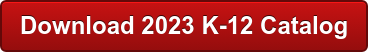Check out these fun geometry facts for kids. Learn about circles, squares, triangles, spheres, cubes and many other interesting shapes!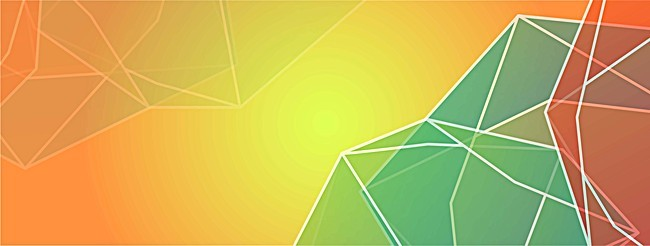## History Facts ...

- The word ‘geometry’ comes from the Greek words ‘geo’, meaning earth, and ‘metria’, meaning measure

- Greek philosopher and mathematician Pythagoras lived around the year 500 BC and is known for his Pythagorean theorem relating to the three sides of a right angle triangle: a² + b² = c²## Triangle Facts...

-The three internal angles of a triangle always add to 180 degrees

-The longest side of a right angle triangle is called the hypotenuse, it is always found opposite the right angle.

-Trigonometry is the study of the relationship between the angles of triangles and their sides.

-Triangle shapes are often used in construction because of their great strength.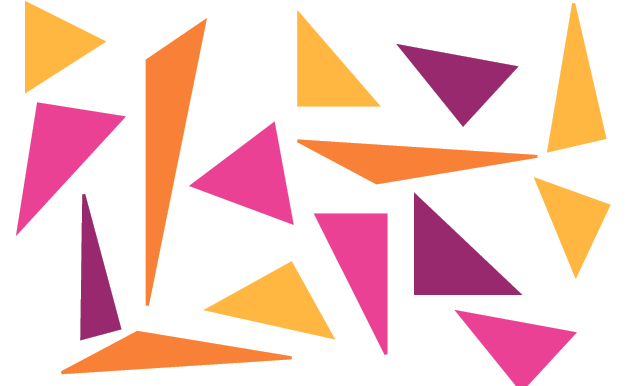## Sphere Facts...

All points on the surface of a sphere are the same distance from the center.

-The distance from the center of a sphere to its surface is called the radius.

-Sometimes a sphere is given a north and south pole (found on opposite sides of the surface).

-A sphere can be divided into two equal hemispheres by a flat two dimensional surface (plane) that passes through the center.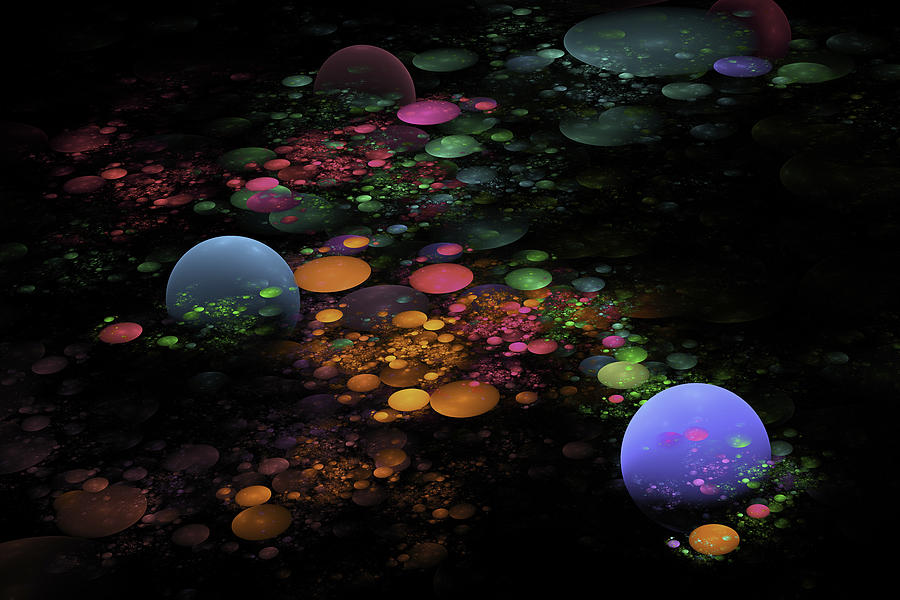## Square Facts...

-A square is also a rectangle with equal sides and a rhombus with right angles.

-The perimeter of a square is 4 times the length of one side.

-The diagonals of a square bisect each other at 90 degrees and are perpendicular.

-The internal angles of a square add to 360 degrees.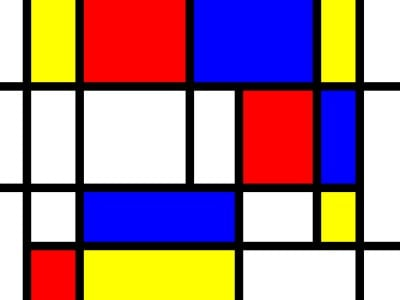## Circle Facts...

-In strict mathematical language, circle refers to the boundary of the shape while ‘disk’ is used to refer to the whole shape, including the inside.

-An arc is part of the circumference of a circle.

-A circle has the shortest perimeter of all shapes with the same area.

-All points on the edge of a circle are the same distance to the center.

-A chord is a straight line joining two points on a circle, the diameter is an example of a chord (the longest possible one).## Curved 3D Shapes Facts...

-A torus is a three dimensional shape that looks like a donut, the inner tube of a tire and a lifebuoy used to help rescue people in water.

-A cone is a three dimensional object with a circular base that narrows toward a point, it looks similar to an ice cream cone.

-A cylinder is a three dimensional object with a circular base and straight parallel sides that looks similar to a can of coke.

-These three dimensional shapes feature curves, which you won't find on polyhedronsCheck more Smart Fridays articles! and visit Engagek12 for amazing lessons with geometry!Check the original article here: http://www.kidsmathgamesonline.com/facts/geometry.html